Next: An example 1-d Poisson Up: Poisson's equation Previous: An example tridiagonal matrix

## 1-d problem with mixed boundary conditions

Previously, we solved Poisson's equation in one dimension subject to Dirichlet boundary conditions, which are the simplest conceivable boundary conditions. Let us now consider the following much more general set of boundary conditions: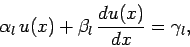(132)

at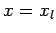, and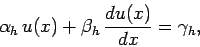(133)

at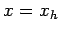. Here,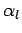,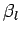, etc., are known constants. The above boundary conditions are termed mixed, since they are a mixture of Dirichlet and Neumann boundary conditions.

Using the previous notation, the discretized versions of Eq. (132) and (133) are: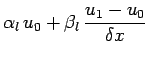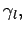(134)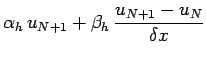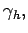(135)

respectively. The above expressions can be rearranged to give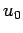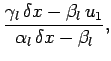(136)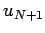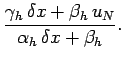(137)

Using Eqs. (115), (136), and (137), the problem can be reduced to a tridiagonal matrix equation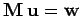, where the left, center, and right diagonals of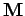possess the elements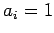for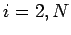, with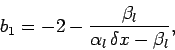(138)

and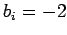for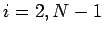, plus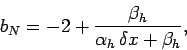(139)

and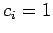for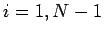, respectively. The elements of the right-hand side are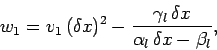(140)

with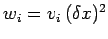for, and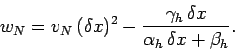(141)

Our tridiagonal matrix equation can be inverted using the algorithm discussed previously.Next: An example 1-d Poisson Up: Poisson's equation Previous: An example tridiagonal matrix
Richard Fitzpatrick 2006-03-29